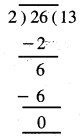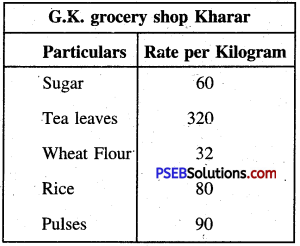# PSEB 5th Class Maths MCQ Chapter 5 Money (Currency)

Punjab State Board PSEB 5th Class Maths Book Solutions Chapter 5 Money (Currency) MCQ Questions and Answers.

## PSEB 5th Class Maths Chapter 5 Money (Currency) MCQ Questions

Multiple Choice Questions

Tick (✓) the right answer :

Question 1.
What is the standard method of writing ₹ 13 and 50 paise ?
(a) ₹ 1350
(b) ₹ 13.50
(c) ₹ 1350 paise
(d) None.
(b) ₹ 13.50

Question 2.
How many ₹ 2 coins are there in ₹ 26?
(a) 52
(b) 26
(c) 13
(d) 20.
(c) 13Question 3.
What is the standard symbol of Indian currency ?
(a) ₹
(b) \$
(c) £
(d) None.
(a) ₹

Question 4.
If the cost of a pen is ₹ 12. What is the cost of 11 pens ?
(a) ₹ 120
(b) ₹ 23
(c) ₹ 1
(d) ₹ 132.
(d) ₹ 132

Question 5.
1 kg apples cost ₹ 80. What is the cost of half kg apples ?
(a) ₹ 20
(b) ₹ 160
(c) ₹ 40
(d) ₹ 80.
(c) ₹ 40Question 6.
The cost of a dozen pencils is ₹ 60. Find the cost of one pencil.
(a) ₹ 12
(b) ₹ 5
(c) ₹ 60
(d) ₹ 30.
(b) ₹ 5

Question 7.
What is the amount of 7 notes of ₹ 20 ?
(a) ₹ 27
(b) ₹ 14
(c) ₹ 140
(d) ₹ 13.
(c) ₹ 140

Question 8.
Convert 480 paise into rupees.
(a) ₹ 4.80
(b) ₹ 48.00
(c) ₹ 480
(d) ₹ 8.40.
(a) ₹ 4.80Question 9.
Sukhdev had spent ₹ 25 in a market and he was left with ₹ 25. How many rupees had he in the beginning ?
(a) ₹ 25
(b) ₹ 5.00
(c) ₹ 50
(d) ₹ 40.
(c) ₹ 50

Question 10.
Find the value of ₹ 10.40 + ₹ 15.30 + ₹ 8.20.
(a) ₹ 33.90
(b) ₹ 34.00
(c) ₹ 30.90
(d) ₹ 339.
(a) ₹ 33.90

Question 11.
The cost of a shirt is ₹ 999.90. How much estimate cost shopkeeper will take ?
(a) ₹ 990
(b) ₹ 999
(c) ₹ 1000
(d) ₹ 999.95
(c) ₹ 1000Question 12.
The cost of a daily newspaper is ₹ 4. What is the cost of newspaper in the month of January ?
(a) ₹ 124
(b) ₹ 12
(c) ₹ 35
(d) ₹ 25.
(a) ₹ 124

Question 13.
Anmol saves ₹ 5 daily from his pocket expenses. How many rupees has he saved in the month of March ?
(a) ₹ 36
(b) ₹ 31
(c) ₹ 155
(d) ₹ 150.
(c) ₹ 155

Question 14.
The cost of 8 meter cloth is ₹ 680. What is the cost of 1 meter cloth ?
(a) ₹ 80
(b) ₹ 85
(c) ₹ 70
(d) ₹ 90.
(b) ₹ 85

Question 15.
How many coins of 50 paise will be there in ₹ 5. ?
(a) 250
(b) 55
(c) 20
(d) 10
(d) 10Question 16.
How many coins of ₹ 2 are there in ₹ 26 ?
₹ 26 ÷ ₹ 2 = 13.Number of ₹ 2 coins in ₹ 26 = 13.

Question 17.
Check the rate list and answer the questions :(i) Cost of 2 kilogram sugar
(ii) Cost of 500 g of tea leaves.
(ii) 500 g = $$\frac{500}{1000}$$ kg = $$\frac{1}{2}$$ kg
Cost of 500 g = $$\frac{1}{2}$$ kg tea leaves
= ₹ 320 × $$\frac{1}{2}$$ = ₹ 160